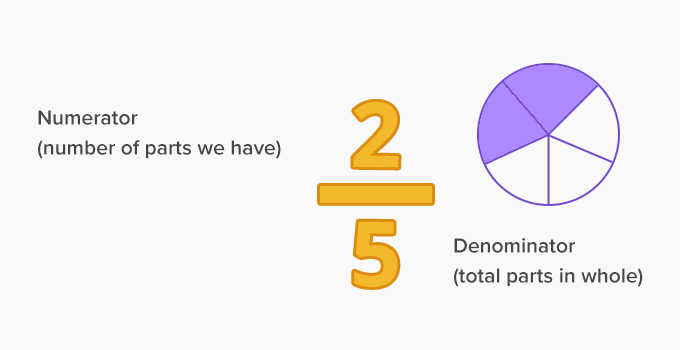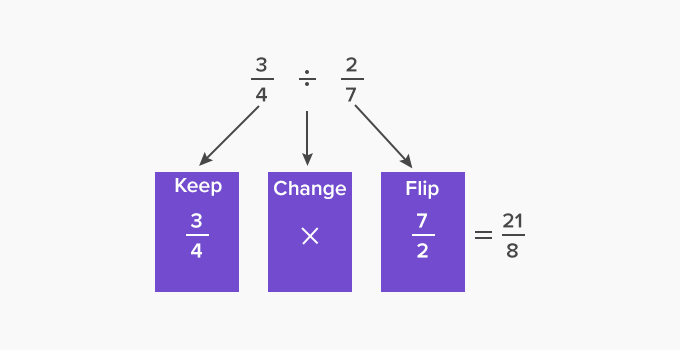# Dividing Fractions - Definition with Examples

The Complete K-5 Math Learning Program Built for Your Child

• 40 Million Kids

Loved by kids and parent worldwide

• 50,000 Schools

Trusted by teachers across schools

• Comprehensive Curriculum

Aligned to Common Core

## Dividing Fractions

A fraction is part of a whole number. It has two parts – a numerator and a denominator.Dividing a fraction

Dividing a fraction by another fraction is the same as multiplying the fraction by the reciprocal (inverse) of the other. We get the reciprocal of a fraction by interchanging its numerator and denominator.

For example, the reciprocal of  25 is52Consider the following example:

1 2 ÷ 1 3

Step 1:

Find the reciprocal of the second fraction (divisor).

Reciprocal of   1 3  is  3 1 or 3

Step 2:

Multiply the first fraction (dividend) by the reciprocal of the second fraction (divisor).

13 x 31

Step 3:

Multiply the numerators and denominators of the fractions.

12 x 31 = 1x32x1 = 32

Dividing Fractions Song

Take the fractions for the division to apply

Flip the second one and then multiply

In the end, you need to simplify.

So next time you divide the fractions don’t forget to apply

The simple rule is to flip and multiply.Dividing a Fraction by a Whole Number

Follow a simple rule that a number divided by 1 is the number itself.

Steps to divide a fraction by a whole number:

• Convert the whole number into a fraction by using a denominator 1.
• Flip this number.
• Multiply by the fraction.
• Simplify the result, if needed.

Take an example, Divide 3by 5

• Convert 5 into a fraction  =51
• Flip 51by  get 15
• Multiply the fractions: 35 x 15 = 325
 Fun-facts  Why do we turn the fraction upside down ?  This is because the inverse operation of division is multiplication.

Won Numerous Awards & Honors